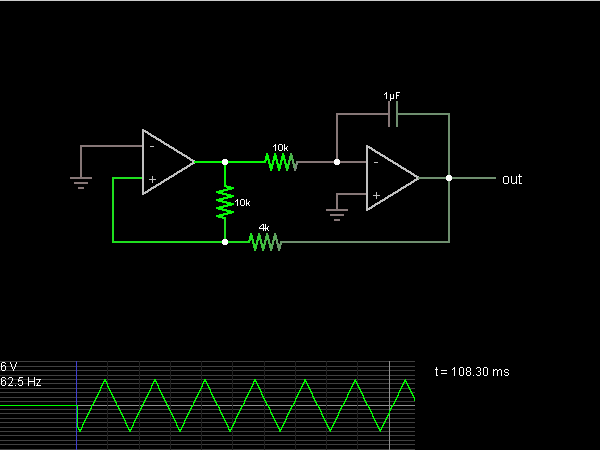# Circuit Simulator - Triangle Wave Generator

Triangle Wave GeneratorCircuit Description:
This circuit is an oscillator that generates a triangle wave.

The second half of the circuit is an inverting integrator. The first op-amp starts with its two inputs in an unknown state; let's say it starts with + slightly higher than – (which is at ground). The op-amp greatly amplifies this difference, bringing its output to the op-amp's positive power supply voltage, its maximum output (15 V in this case). With this positive input, the integrator's output falls at a constant rate.

The 10k and 4k resistors act as a voltage divider which put the first op-amp's + input 4/14ths of the way from the second op-amp's output to the first op-amp's output. When this input reaches ground, then the first op-amp's output switches polarity, and the integrator switches direction, forming the other half of the triangle. When the first op-amp switches polarity again, a new cycle begins.

Discussion:
1 comments Page 1 of 1.

Yi wen sau said:   8 years ago
Then how do I generate 40 kHz triangle wave? Thanks.
(4)